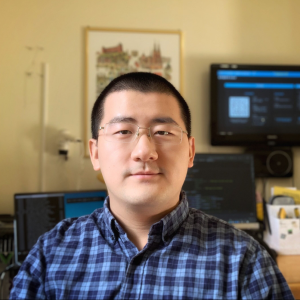# Natural Computation Methods for Machine Learning Note 11

2020年3月4日 2967点热度 0人点赞 0条评论

# Natural Computation Methods for Machine Learning Note 11

Let us firstly revisit completive learning and SOFM

## Review

### CL and SOFM

• A node position (in the input space) is its weight vector, i.e. nodes move around in the same space as the data.
• Purpose of unsupervised learning is (usually) to cluster for classification, or for modelling distributions (several nodes in high density areas, fewer in other areas)

### CL with bad (random) initialization (simulation)

• The winner-takes-all scenario
• Nodes which are never used (= no data in that Voronoi region)
• Nodes which cover more than one cluster, and therefore moves back and
forth between them

Nodes moving back and forth between clusters = nodes that need help? Node "instability" as an error measure ?

### CL initialized from the data (simulation)

• Better coverage. All nodes are used.
• But still not optimal, unless we're very lucky. Bad distribution of nodes.

### Rectangular distribution example (simulation)

What should we expect if we run CL with 10 nodes on this distribution? What if the distribution moves, slowly. What if it jumps?

SOFM on the same data. SHOW gridlines. Should follow jumps, were it not for the decaying parameters

### SOFM (simulation)

SOFM forms a density function of the data. For example, uniformly distributed input data leads to uniformly distributed weight vectors. Three steps:

Random. Edges join nodes that are neighbours on the map.

A fishing net, since neighbours in the network, the grid, tend to react on the same data (topological map).

A stretched net, as the nodes move towards the different clusters.

Should two such nodes still be neighbours? Is it possible to have a dynamic neighbourhood, where edges can be created and destroyed? Rubber bands that snap?

## Growing Neural Gas

Unsupervised growing algorithm for clustering.

• Dynamic size – a growing/shrinking algorithm
• though it grows faster than it shrinks
• Dynamic neighbourhood – who is neighbour to whom is not fixed
• defined by a graph (SHOW). Not weighted connections, just edges in a graph! (like the SOFM grid lines).
• All parameters are constant
• great for on-line learning and for following moving targets
• GNG will even follow jumping targets

Three things to consider:

• Which nodes to move (given an input), and by how much
• How to define and update the neighbourhood graph
• How to grow (when and where should we insert new nodes)

### Which nodes to move

Move not only the winner (k), but also its (current) neighbours. The winner is moved using a much greater gain factor than the neighbours:
\begin{aligned}
&\Delta w_{k}=\varepsilon_{k}\left(x-w_{k}\right)\
&\Delta w_{n}=\varepsilon_{n}\left(x-w_{n}\right)^{, \boldsymbol{\varepsilon}_{k}>>} \varepsilon_{n}
\end{aligned}

where k is the winner, n is a neighbour, x is the input vector and w is the node position (its weight vector). Both gain factors are constant. (note that n in the equations is a name, not an index)

### Neighbourhood (and shrinking)

All current neighbours are connected by edges in a graph.
Each edge has an associated age

For each input vector

1. find the the closest node (k, the winner), and the second closest (r).
2. If k and r are not already connected (neighbours), connect them with a new edge
3. Set the age of the edge between k and r to 0.
4. The age of all other edges from k is incremented by one.
• If any edge becomes too old (> amax), remove it. If any node loses its last edge this way, remove that node as well.

### Growth

Every time a winner, k, is found, add the distance (from the input) to a local error variable.

(error is proportional to the accumulated distance this node as moved, as a winner)

At fixed time intervals, insert a new node where it is most likely needed:

• halfway between the node with the largest error, and the node among its current neighbours with the largest error.

The error of a node is decayed over time (this is not a decaying parameter, though)

See the algorithm in Fritzkes paper (in the handouts)
Possible problem: Dead units (show using a jumping rectangular distribution) – could be an advantage, though

Extension: GNG-U. Removes nodes with low utility, based on frequency of winning and closeness to other nodes.

Show Delaunay triangulation and that GNG forms a subset of this.

GNG is used mostly for modelling distributions. Can for example be used to train the hidden nodes in RBF networksDong Wang

I will work as a PhD student of TU Graz in Austria. My research interests include Embedded/Edge AI, federated learning, computer vision, and IoT.